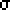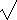# Power and Sample Size

#### Lesson Overview

The role of sample size in the power of a statistical test must be considered before we go on to advanced statistical procedures such as analysis of variance/covariance and regression analysis. One can select a power and determine an appropriate sample size beforehand or do power analysis afterwards. However, power analysis is beyond the scope of this course and predetermining sample size is best.

### Sample Size Importance

 An appropriate sample size is crucial to any well-planned research investigation.

Although crucial, the simple question of sample size has no definite answer due to the many factors involved. We expect large samples to give more reliable results and small samples to often leave the null hypothesis unchallenged. Large samples may be justified and appropriate when the difference sought is small and the population variance large. Established statistical procedures help ensure appropriate sample sizes so that we reject the null hypothesis not only because of statistical significance, but also because of practical importance. These procedures must consider the size of the type I and type II errors as well as the population variance and the size of the effect. The probability of committing a type I error is the same as our level of significance, commonly, 0.05 or 0.01, called alpha, and represents our willingness of rejecting a true null hypothesis. This might also be termed a false negative—a negative pregnancy test when a woman is in fact pregnant. The probability of committing a type II error or beta (ß) represents not rejecting a false null hypothesis or false positive—a positive pregnancy test when a woman is not pregnant. Ideally both types of error are minimized. The power of any test is 1 - ß, since rejecting the false null hypothesis is our goal.

### Power of a Statistical Test

 The power of any statistical test is 1 - ß.

Unfortunately, the process for determining 1 - ß or power is not as straightforward as that for calculating alpha. Specifically, we need a specific value for both the alternative hypothesis and the null hypothesis since there is a different value of ß for each different value of the alternative hypothesis. Fortunately, if we minimize ß (type II errors), we maximize 1 - ß (power). However, if alpha is increased, ß decreases. Alpha is generally established before-hand: 0.05 or 0.01, perhaps 0.001 for medical studies, or even 0.10 for behavioral science research. The larger alpha values result in a smaller probability of committing a type II error which thus increases the power.

Example: Suppose we have 100 freshman IQ scores which we want to test a null hypothesis that their one sample mean is 110 in a one-tailed z-test with alpha=0.05. We will find the power = 1 - ß for the specific alternative hypothesis of IQ>115.
Solution: Power is the area under the distribution of sampling means centered on 115 which is beyond the critical value for the distribution of sampling means centered on 110. More specifically, our critical z = 1.645 which corresponds with an IQ of 1.645 = (IQ - 110)/(15/sqrt(100)) or 112.47 defines a region on a sampling distribution centered on 115 which has an area to the right of z = -1.69 or 0.954. Note that we have more power against an IQ of 118 (z= -3.69 or 0.9999) and less power against an IQ of 112 (z = 0.31 or 0.378).

The basic factors which affect power are the directional nature of the alternative hypothesis (number of tails); the level of significance (alpha); n (sample size); and the effect size (ES). We will consider each in turn.

Example: Suppose we change the example above from a one-tailed to a two-tailed test.
Solution: We first note that our critical z = 1.96 instead of 1.645. There are now two regions to consider, one above 1.96 = (IQ - 110)/(15/sqrt(100)) or an IQ of 112.94 and one below an IQ of 107.06 corresponding with z = -1.96. Most of the area from the sampling distribution centered on 115 comes from above 112.94 (z = -1.37 or 0.915) with little coming from below 107.06 (z = -5.29 or 0.000) for a power of 0.915. For comparison, the power against an IQ of 118 (below z = -7.29 and above z = -3.37) is 0.9996 and 112 (below z = -3.29 and above z = 0.63) is 0.265.

 One-tailed tests generally have more power.

Example: Suppose we instead change the first example from alpha=0.05 to alpha=0.01.
Solution: Our critical z = 2.236 which corresponds with an IQ of 113.35. The area is now bounded by z = -1.10 and has an area of 0.864. For comparison, the power against an IQ of 118 (above z = -3.10) is 0.999 and 112 (above z = 0.90) is 0.184.

 "Increasing" alpha generally increases power.

Since a larger value for alpha corresponds with a small confidence level, we need to be clear we are referred strictly to the magnitude of alpha and not the increased confidence we might associate with a smaller value!

Example: Suppose we instead change the first example from n = 100 to n = 196.
Solution: Our critical z = 1.645 stays the same but our corresponding IQ = 111.76 is lower due to the smaller standard error (now 15/14 was 15/10). Our z = -3.02 gives power of 0.999. For comparison, the power against an IQ of 118 (above z = -5.82) is 1.000 and 112 (above z = -0.22) is 0.589.

 Increasing sample size increases power.

For comparison we will summarize our results:

factors\Ha=112115118
1-tail, alpha=0.05, n = 100 0.378 0.954 0.9999
2-tail, alpha=0.05, n = 100 0.265 0.915 0.9996
1-tail, alpha=0.01, n = 100 0.184 0.864 0.9990
1-tail, alpha=0.05, n = 196 0.589 0.999 1.0000

When one reads down the columns in the table above, we show the affect of the number of tails, the value of alpha, and the size of our sample on power. When one reads across the table above we see how effect size affects power.

 A statistical test generally has more power against larger effect size.

We should note, however, that effect size appears in the table above as a specific difference (2, 5, 8 for 112, 115, 118, respectively) and not as a standardized difference. These correspond to standardized effect sizes of 2/15=0.13, 5/15=0.33, and 8/15=0.53.

 The process of determining the power of the statistical test for a two-sample case is identical to that of a one-sample case. Exactly the same factors apply.

Hinkle, page 312, in a footnote, notes that for small sample sizes (n < 50) and situations where the sampling distribution is the t distribution, the noncentral t distribution should be associated with Ha and the power calculation. Formulas and tables are available or any good statistical package should use such.

### Sample Size Calculations

It is considered best to determine the desired power before establishing sample size rather than after.

 Some behavioral science researchers have suggested that Type I errors are more serious than Type II errors and a 4:1 ratio of ß to alpha can be used to establish a desired power of 0.80.

Using this criterion, we can see how in the examples above our sample size was insufficient to supply adequate power in all cases for IQ = 112 where the effect size was only 1.33(for n = 100) or 1.87.

We now have the tools to calculate sample size. We start with the formula z = ES/(/n) and solve for n. The z used is the sum of the critical values from the two sampling distribution. This will depend on alpha and beta.

Example: Find z for alpha=0.05 and a one-tailed test.
Solution: We would use 1.645 and might use -0.842 (for a ß = 0.20 or power of 0.80).

Example: For an effect size (ES) above of 5 and alpha, beta, and tails as given in the example above, calculate the necessary sample size.
Solution: Solving the equation above results in n =2z2/(ES)2 = 152 • 2.4872 / 52 = 55.7 or 56. Thus in the first example, a sample size of only 56 would give us a power of 0.80.

Note: it is usual and customary to round the sample size up to the next whole number. Thus pi=3.14... would round up to 4.

Example: Find the minimum sample size needed for alpha=0.05, ES=5, and two tails for the examples above.
Solution: The necessary z values are 1.96 and -0.842 (again)---we can generally ignore the miniscule region associated with one of the tails, in this case the left. The same formula applies and we obtain: n = 225 • 2.8022 / 25 = 70.66 or 71.

 For a given effect size, alpha, and power, a larger sample size is required for a two-tailed test than for a one-tailed test.

Recalling the pervasive joke of knowing the population variance, it should be obvious that we still haven't fulfilled our goal of establishing an appropriate sample size. There are two common ways around this problem. First, it is acceptable to use a variance found in the appropriate research literature to determine an appropriate sample size. Second, it is also common to express the effect size in terms of the standard deviation instead of as a specific difference. Since effect size and standard deviation both appear in the sample size formula, the formula simplies.

Tables to help determine appropriate sample size are commonly available. Such tables not only address the one- and two-sample cases, but also cases where there are more than two samples. Since more than one treatment (i.e. sample) is common and additional treatments may reduce the effect size needed to qualify as "large," the question of appropriate effect size can be more important than that of power or sample size. That question is answered through the informed judgment of the researcher, the research literature, the research design, and the research results.

We have thus shown the complexity of the question and how sample size relates to alpha, power, and effect size.

 Effect size, power, alpha, and number of tails all influence sample size.